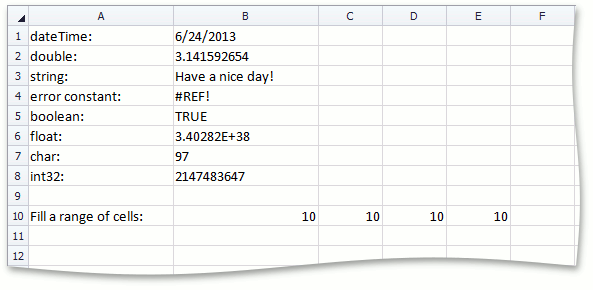21.1
20.2
20.1
19.2
19.1
18.2
18.1
17.2
A newer version of this page is available. Switch to the current version.

# How to: Change a Cell or Cell Range Value

• 2 minutes to read

This example demonstrates how to use the Range.Value property to add data of different types to worksheet cells.

Enclose your code in the SpreadsheetControl.BeginUpdate - SpreadsheetControl.EndUpdate method calls to suppress the SpreadsheetControl’s visual updates and improve its performance when you edit numerous cells in a document.

##### NOTE

A complete sample project is available at https://github.com/DevExpress-Examples/winforms-spreadsheetcontrol-api-e4655

``````// Add data of different types to cells.
worksheet.Cells["B1"].Value = DateTime.Now;
worksheet.Cells["B2"].Value = Math.PI;
worksheet.Cells["B3"].Value = "Have a nice day!";
worksheet.Cells["B4"].Value = CellValue.ErrorReference;
worksheet.Cells["B5"].Value = true;
worksheet.Cells["B6"].Value = float.MaxValue;
worksheet.Cells["B7"].Value = 'a';
worksheet.Cells["B8"].Value = Int32.MaxValue;

// Fill all cells in the range with 10.
worksheet.Range["B10:E10"].Value = 10;
``````

The following image shows how data of different types are displayed in cells:## Convert a String to a Cell Value

The example below demonstrates how to use the Range.SetValueFromText method to automatically convert a specified string to a CellValue object of the appropriate data type and assign it to a cell. Set the method’s preserveNumberFormat parameter to true to retain the cell’s Formatting.NumberFormat.

``````// Add data of different types to cells.
worksheet.Cells["B1"].SetValueFromText("28-Jul-20 5:43PM"); // DateTime
worksheet.Cells["B2"].SetValueFromText("3.1415926536"); // double
worksheet.Cells["B3"].SetValueFromText("Have a nice day!"); // string
worksheet.Cells["B4"].SetValueFromText("#REF!"); // error
worksheet.Cells["B5"].SetValueFromText("true"); // Boolean
worksheet.Cells["B6"].SetValueFromText("3.40282E+38"); // float
worksheet.Cells["B7"].SetValueFromText("2147483647"); // int32
worksheet.Cells["B8"].NumberFormat = "d-mmm-yy h:mm";
worksheet.Cells["B8"].SetValueFromText("28-Jul-20 5:43PM", true); // DateTime with a custom format
worksheet.Cells["B9"].SetValueFromText("=SQRT(25)"); // formula
``````

If you need to assign an object of any type to a cell value, use the Range.SetValue method as shown in the How to: Convert Objects to Cell Values and Cell Values to Objects example.

See Also